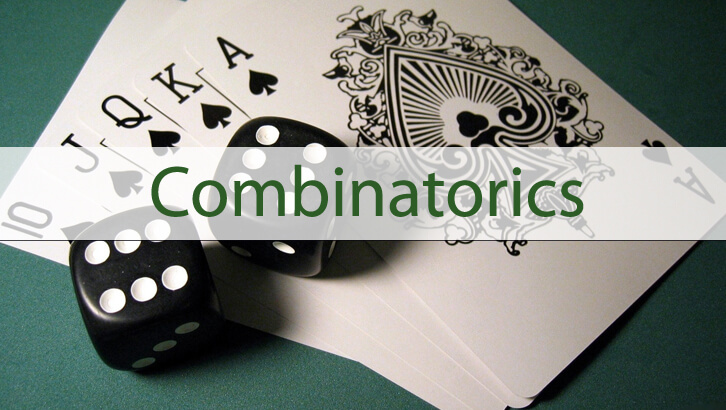# CAT – Probability

### Probability Question

Question:
[x] = greatest integer less than equal to x. If x lies between 3 and 5. What is the probability than [x2] = [x]2?
A. Roughly 0.64
B. Roughly 0.5
C. Roughly 0.14
D. Roughly 0.36
Correct Answer: (C)
Explanation:
Let us take a few examples.
 = 2
[3.52] =12             [3.5]2 = 9
 = 16               2 = 16
For x Î (3, 5). [x]2 can only take value 9, 16 and 25.
Let us see when [x2] will be 9, 16 or 25
If [x2] = 9,  x2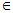[9, 10)
=> x[3,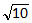)
[x2] = 16 => x2[16, 17)
=> x[4,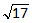)
In the given range [x2] = 25 only when x = 5
So [x2] = [x]2 when x[3,] or [4,) or 5.
Probability =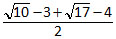=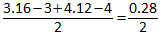= 0.14
Difficulty Level 2